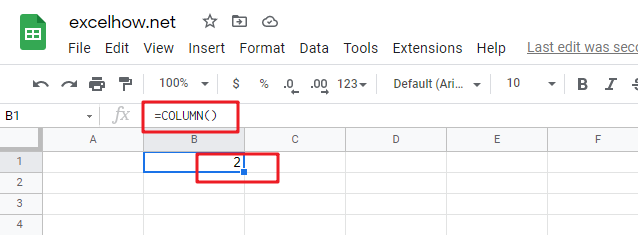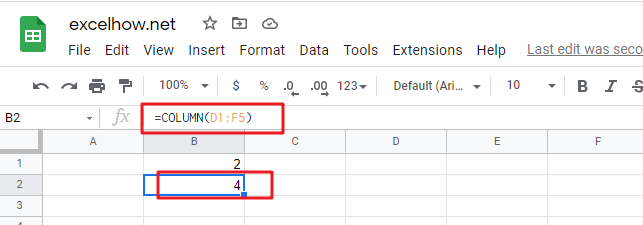# ExcelHow

This post will guide you how to use Google Sheets COLUMN function with syntax and examples.

## Description

The Google Sheets COLUMN function returns the first column number of the given cell reference. For example, COLUMN(B4) returns 2, as C is the second column in the google sheets.

The COLUMN function can be used to get the column number for a cell reference in google sheets. The purpose of this function is to get the column number of a reference and the returned value is a number that representing the column.

The COLUMN function is a build-in function in Google Sheets and it is categorized as a Lookup function.

## Syntax

The syntax of the COLUMN function is as below:

=COLUMN ([reference])

Where the COLUMN function arguments are:

• Reference -This is an optional argument. A reference to a cell or a range of cells for which you want to get the first column number.

Note:

• If the Array argument is omitted, the COLUMN function will return the column number of the cell that the function is entered in
• COLUMN function only takes one argument, it can be a cell reference, a cell range.
• COLUMN function can not contain multiple cell reference.

## Google Sheets COLUMN Function Examples

The below examples will show you how to use google sheets COLUMN Function to return the column number of a cell reference.

#1 To get the number of the column in B1 Cell, just using the following COLUMN formula:

`=COLUMN ( )`#2 To get the number of column in the reference D1:F5, just using the following formula:

`=COLUMN(D1:F5)`